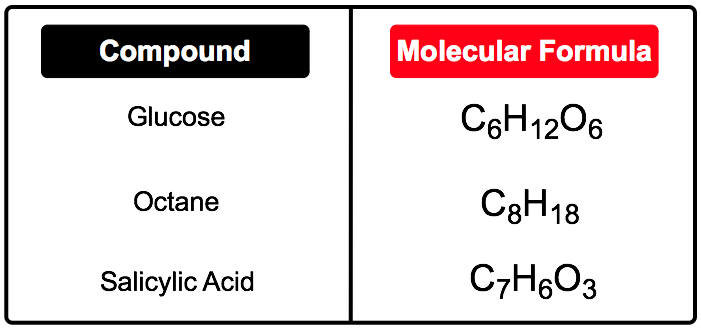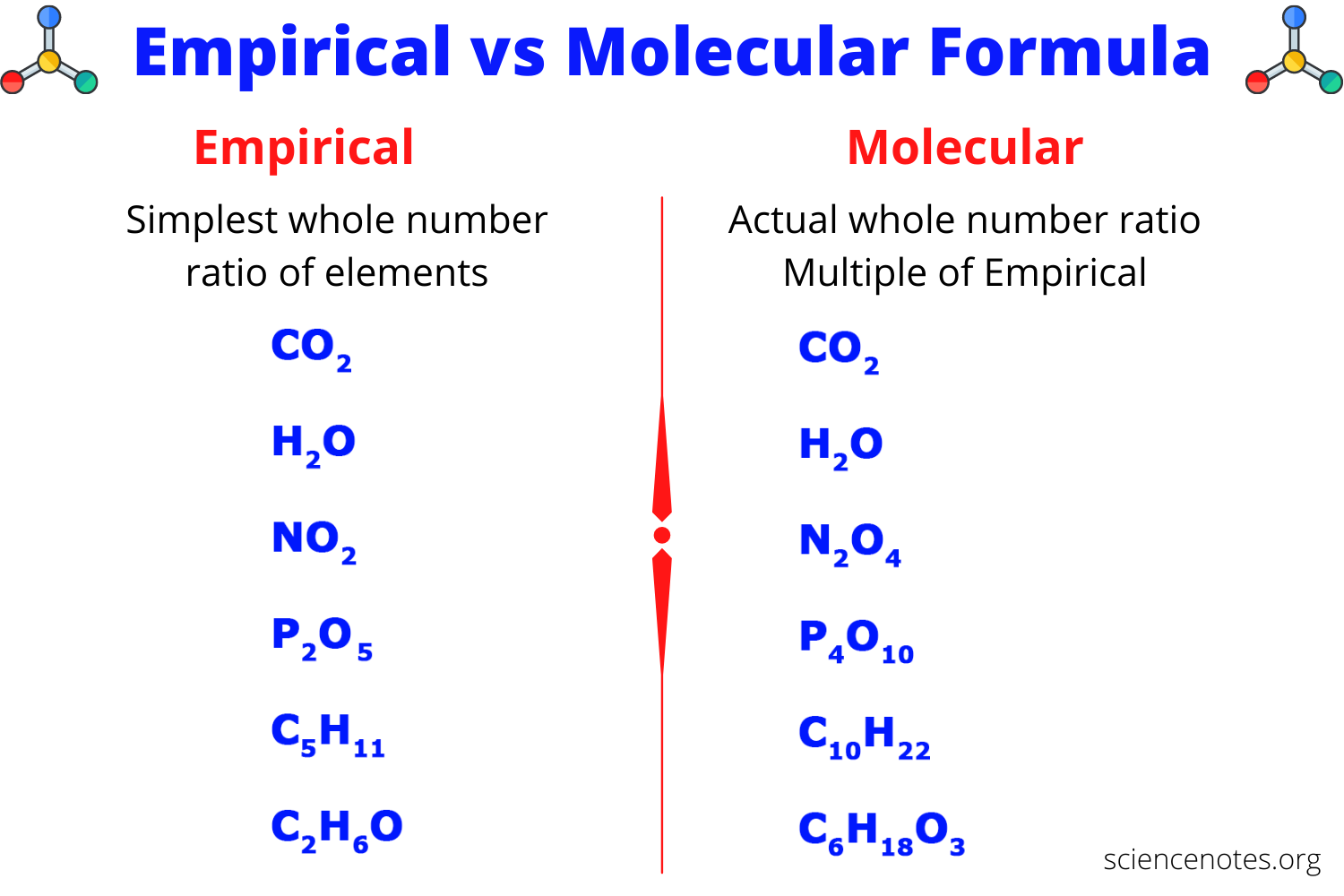Crystal

HS-PS1-1

# Molecular Formula And Its Calculations Study Guide

The molecular formula is an expression that helps to determine the number and type of atoms present in a molecule of a substance. One molecular formula example is CO₂.

## INTRODUCTION

Have you ever wondered by looking at a chemical reaction how it is determined? The big formulas or images will look complex, but if you know the molecular formula, it becomes very simple and easy to understand. Now, the first thing that will strike you is what is a molecular formula, and how is it helpful to understand the different yet complex chemical reactions? Let us dig into it!

### WHAT IS THE MOLECULAR FORMULA?

Before understanding the molecular formula, do you know what a molecule is? A molecule is a chemical combination of two atoms. The molecular formula of a molecular compound is a chemical formula that shows the number of different atoms present in a molecule. For example, in CO₂, the subscript under oxygen shows that there are two atoms of oxygen present, whereas the lack of subscript under carbon shows it has only one atom of carbon present.

The chemical formula can be written as:

Molecular formula = n (Empirical formula)

where n = integer multiple

It can be also written as:

n = MM (molar mass) / EFM (empirical formula molar mass)Source

### WHAT IS THE EMPIRICAL FORMULA?

The empirical formula of a compound is the simplest whole-number ratio of atoms. It can be easily determined from the percent composition of a compound.Source

### HOW TO FIND A MOLECULAR FORMULA FROM THE EMPIRICAL FORMULA?

We will need to know the empirical formula so that we can convert it to a molecular formula. You also need to know the molar mass of a compound to get the molecular formula. Here are simple steps to determine the molecular formula:

Step 1: Calculate the empirical formula molar mass (EFM).

Step 2: You need to divide the molar mass of the compound by the empirical formula molar mass (EFM). The result should be a whole number or very close to a whole number.

Step 3: You have to multiply all the subscripts in the empirical formula by the whole number found. The result that we will get is the molecular formula.

### EXAMPLE PROBLEM TO FIND OUT THE MOLECULAR FORMULA

The empirical formula of a compound of boron and hydrogen is BH3. Its molar mass is 27.7g/mol. Determine the molecular formula of the compound.

Given: Empirical formula = BH₃

``````     Molar mass = 27.7 g/mol
``````

Empirical formula molar mass (EFM) = 13.84 g/mol

Molar mass / EFM = 27.7 g/mol / 13.84 g/mol = 2

The molecular formula = BH₃ × 2 = B₂H₆

### FAQs:

1. What is the molecular formula short answer?

It is a chemical formula that helps determine the total number of atoms present in a molecule.

2. What is the empirical and molecular formula, with an example?

The empirical formula of a compound is the simplest whole-number ratio of atoms, whereas the molecular formula is the total number of atoms present in a molecule. An example of the empirical formula is hexane is C₃H₇, and the molecular formula is CO₂.

3. How do you find the molecular formula?

Molecular Formula = n (Empirical formula)

where n = integer multiple

4. What is a mole? Explain with an example?

It is defined as the mass of a substance containing 6.023 x 10²³ particles of a substance. Example: In 12 gms carbon, there are 12 x 6.023 x 10²³ moles present.

We hope you enjoyed studying this lesson and learned something cool about the Molecular Formula and its Calculations! Join our Discord community to get any questions you may have answered and to engage with other students just like you! Don't forget to download our app to experience our fun VR classrooms - we promise it makes studying much more fun! 😎

## SOURCES

1. Molecular Formula: https://flexbooks.ck12.org/cbook/ck-12-chemistry-flexbook-2.0/section/7.1/primary/lesson/molecular-formula-chem/. Accessed 28 Feb 2022.
2. Empirical Formula: https://flexbooks.ck12.org/cbook/ck-12-chemistry-flexbook-2.0/section/7.2/primary/lesson/empirical-formula-chem/. Accessed 28 Feb 2022.